150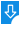10
2585

# Logic Calculator  (1.0 Beta) Free Download

Logic Calculator 1.0 Beta Description:
Logic Calculator is a self-explanatory OS X application that provides the necessary tools and features to perform various logical operations. From Logic Calculator's main window you will be able to access three different modes, the Evaluation of the logic formula, Logical entailment and Conversion of a formula to Disjunctive Normal Form (DNF) and Conjunctive Normal Form (CNF). In the first mode you can easily use the basic boolean operations such as negation, disjunction, conjunction, biconditional, conditional and insert them in the logic formula. Logic Calculator will output the truth table and the models of the formula.Logic Calculator: Logic Calculator for Mac, A free and open-source calculator specially designed to help you perform a wide variety of logical operation from within a user-friendly interface
Publisher: Oscar Ch?vezDownload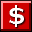Buy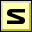Screenshot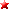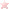Rate it
Category: Math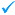Publisher: Oscar Ch?vez    More titles >>
Last Updated: 03/09/2019
Requirements: Mac OS X